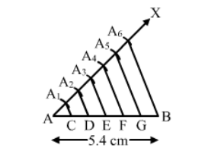# Draw a line segment AB of length 5.4 cm.`
Question:

Draw a line segment AB of length 5.4 cm. Divide it into six equal parts. Write the steps of construction.

Solution:

Steps of Construction :

Step 1 . Draw a line segment AB = 5.4 cm.

Step 2. Draw a ray $A X$, making an acute angle, $\angle B A X$.

$\mathrm{AA}_{1}=\mathrm{A}_{1} \mathrm{~A}_{2}=\mathrm{A}_{2} \mathrm{~A}_{3}=\mathrm{A}_{3} \mathrm{~A}_{4}=\mathrm{A}_{4} \mathrm{~A}_{5}=\mathrm{A}_{5} \mathrm{~A}_{6}$.

Step 4. Join A6B.
Step 5. Draw A1A2D, A3D, A4F and A5G .Thus, AB is divided into six equal parts.